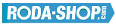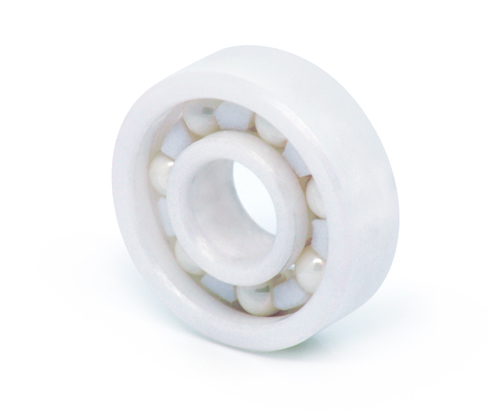# Hybrid and Ceramic BearingsManufacturer Product Image Item Name Price### 6007-CE-ZR02-PFTE

Solid Ceramic Bearings Ceramic Open(No Shields) Dimension d=35 Dimension D=62 Dimension B=14 Dinamic Load Rating=9600 Static Load Rating=6180 Weight=0.151gr. Speed limit Grease=N/A Speed limit Oil=0 Flanged
inside diameter = 35
outside diameter = 62
width = 14
call### 6008-CE-SI3N4-PFTE

Solid Ceramic Bearings Ceramic Open(No Shields) Dimension d=40 Dimension D=68 Dimension B=15 Dinamic Load Rating=10080 Static Load Rating=6900 Weight=0.19gr. Speed limit Grease=N/A Speed limit Oil=0 Flanged
inside diameter = 40
outside diameter = 68
width = 15### 6008-CE-ZR02-PFTE

Solid Ceramic Bearings Ceramic Open(No Shields) Dimension d=40 Dimension D=68 Dimension B=15 Dinamic Load Rating=10080 Static Load Rating=6900 Weight=0.19gr. Speed limit Grease=N/A Speed limit Oil=0 Flanged
inside diameter = 40
outside diameter = 68
width = 15
call### 608-CE-SI3N4-PFTE

Solid Ceramic Bearings Ceramic Open(No Shields) Dimension d=8 Dimension D=22 Dimension B=7 Dinamic Load Rating=1976 Static Load Rating=827 Weight=0.014gr. Speed limit Grease=N/A Speed limit Oil=0 Flanged
inside diameter = 8
outside diameter = 22
width = 7### 608-CERAMICO-ZR02-PFTE

Solid Ceramic Bearings Ceramic Open(No Shields) CAGE-PTFE Dimension d=8 Dimension D=22 Dimension B=7 Dinamic Load Rating= Static Load Rating= Weight=0.014gr. Speed limit Grease=N/A Speed limit Oil=0
inside diameter = 8
outside diameter = 22
width = 7
call### 609-CE-SI3N4-PFTE

Solid Ceramic Bearings Ceramic Open(No Shields) Dimension d=9 Dimension D=24 Dimension B=7 Dinamic Load Rating=2014 Static Load Rating=866 Weight=0.016gr. Speed limit Grease=N/A Speed limit Oil=0 Flanged
inside diameter = 9
outside diameter = 24
width = 7### 609-CE-ZR02-PFTE

Solid Ceramic Bearings Ceramic Open(No Shields) Dimension d=9 Dimension D=24 Dimension B=7 Dinamic Load Rating=2014 Static Load Rating=866 Weight=0.016gr. Speed limit Grease=N/A Speed limit Oil=0 Flanged
inside diameter = 9
outside diameter = 24
width = 7
call### 61903-CE-SI3N4-PFTE

Solid Ceramic Bearings Ceramic Open(No Shields) Dimension d=17 Dimension D=30 Dimension B=7 Dinamic Load Rating=2753 Static Load Rating=1539 Weight=0.018gr. Speed limit Grease=N/A Speed limit Oil=0 Flanged
inside diameter = 17
outside diameter = 30
width = 7
€65.34### 6200-CE-SI3N4-PFTE

Solid Ceramic Bearings Ceramic Open(No Shields) Dimension d=10 Dimension D=30 Dimension B=9 Dinamic Load Rating=3060 Static Load Rating=1434 Weight=0.032gr. Speed limit Grease=N/A Speed limit Oil=0 Flanged
inside diameter = 10
outside diameter = 30
width = 9### 6200-CE-ZR02-PFTE

Solid Ceramic Bearings Ceramic Open(No Shields) Dimension d=10 Dimension D=30 Dimension B=9 Dinamic Load Rating=3060 Static Load Rating=1434 Weight=0.032gr. Speed limit Grease=N/A Speed limit Oil=0 Flanged
inside diameter = 10
outside diameter = 30
width = 9
call